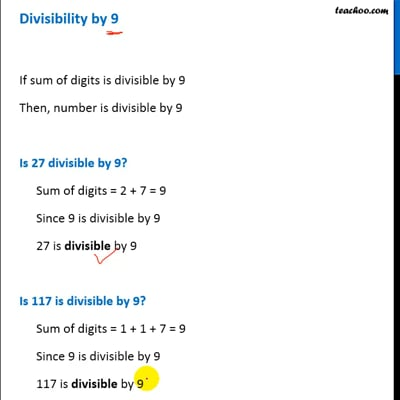Divisibility Tests - Divisibility by 3, 6, 9

Chapter 3 Class 6 Playing with Numbers
Concept wise

If sum of digits is divisible by 9

Then, number is divisible by 9

#### Is 27 divisible by 9?

Sum of digits = 2 + 7 = 9

Since 9 is divisible by 9

27 is divisible by 9

#### Is 117 is divisible by 9?

Sum of digits = 1 + 1 + 7 = 9

Since 9 is divisible by 9

117 is divisible by 9

#### Is 758 divisible by 9?

Sum of digits = 7 + 5 + 8 = 20

Since 20 is not divisible by 9

So, 758 is not divisible by 9This video is only available for Teachoo black users